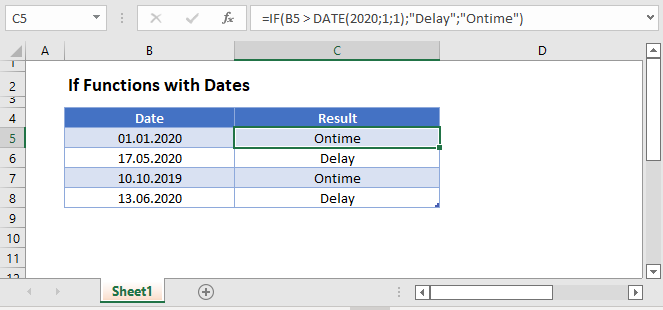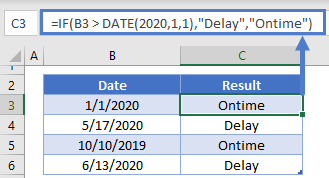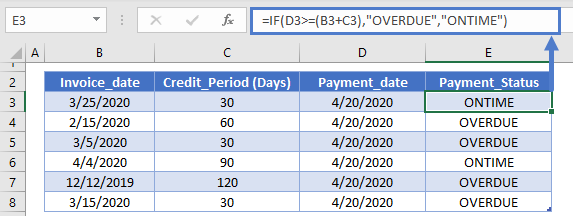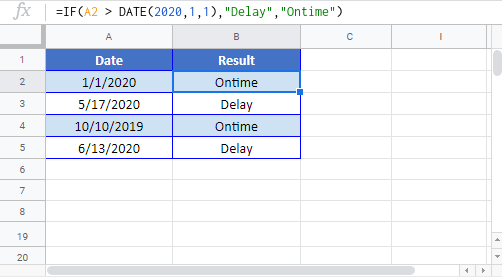# If Functions with Dates – Excel & Google Sheets

This tutorial will demonstrate how to use the IF Function with Dates in Excel and Google Sheets.## IF & DATE Functions

To use dates within IF Functions, you can use the DATE Function to define a date:

``=IF(B3 > DATE(2020,1,1),"Delay","Ontime")``One example of this formula is to calculate if a payment is over due:

### Payment Over Due

``=IF(D3>=(B3+C3),"OVERDUE","ONTIME")``## If Function with Dates – Google Sheets

All of the above examples work exactly the same in Google Sheets as in Excel.### Excel Practice Worksheet

Practice Excel functions and formulas with our 100% free practice worksheets!